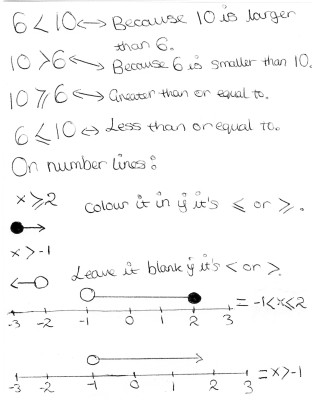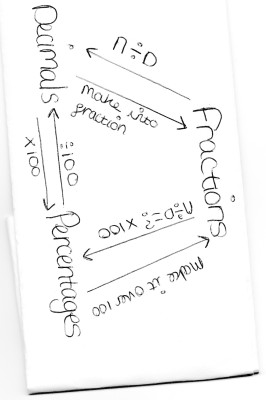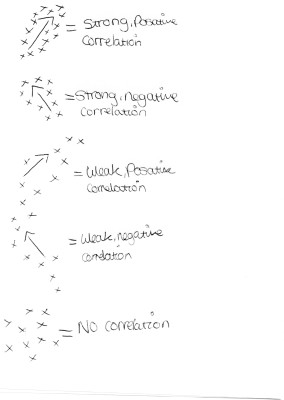List of public pages created with Protopage

# Home

## Plain sticky notes

### Transformations

TRANSFORMATIONS: 1.Translation uses vectors (7) top means right or if its a minus number left (3) bottom means up or if its a minus number down 2.Enlargement uses: a. Scale Factor b.Centre Of Enlargement 3.Reflection a.uses a mirror line 4.Rotation uses: a.Centre Of Rotation b.Degrees/angles c.Direction

### How to calculate a fraction

1. Divide the denominator 2.Multiply the numerator eg. 2/3 out of 12 12 divided by 3 =4 x2 =8 hint: numerator is the top, denominator is the bottom!!

### Linear Graphs

The general equasion for a straight line is: Y=mx+c The m and c can be any number The m stands for the GRADIENT (steepness) The c stands for the Y INTERCEPT (where the graph crosses the Y axis) Rise over run: If you are asked to describe a linear graph you would use Rise over Run You would use this when you have drawn the triangle (check video!!!) The rise is how many squares up. The run is how many squares along A way to remember "along the corridoor up the stairs" For example Rise= 4 Run=2 4 divided by 2=2 So this is the gradient and where it crosses the graph is the y-intercept so... y=mx+c m=2 c=-1 (for example) y=2x-1 A substitution grid is used with linear graphs: X -3 -2 -1 0 1 2 3 Y=3x+1 -10 -7 -4 -1 2 5 8 These are the points you would plot onto a linear graph. The rule is y=3x+1 so you do 3 times the number in the top row (X) Plus 1 which gives you your number to plot Hint: the number (m-gradient 3 in my example) shows you the difference there should be between each number if it's not that number something has gone wrong.

### Times fractions

1. Times the top numbers 2. Times the bottom numbers 2 x 4 = 8 3 7 21

### dividing fractions

To divide a fraction you have to look at the 2nd fraction and flip it upside down for example 2/3 divided by 8/9 gets flipped into 2/3 divided by 9/8 then you times the numerators and the denominators. 2/3 x 9/8 =18/24

### Percentage of an amount

Find 20% of £300 300 divided by 100 is 3 3 multiplied by 20 is 60 1. Divide the total amount by 100. 2. Multiply by the percentage So your answer is 60.

### Ratio

eg. 5:7 of 96 1. Add the ratio 5+7=12 2. Divide total amount by answer in step 1 96 divided 12=8 3. Multiply answer of step 2 by each part of ratio 8x5=40 8x7=56 4. Put it in ratio form 40:56

### MMMR

Mean- Add all together and divide by the amount of numbers Median- The middle number Mode- The most common number Range- The difference between the largest and the smallest number

### Numbers in words

Quite simple really just a little help on how you write numbers. 1823.15 One thousand eight hundred and twenty three point one five Hint: you only ever add "and" in when you would actually say it. You could get marks taken off in the exam!!!!!

### Times Tables

1x: 1,2,3,4,5,6,7,8,9,10,11,12 2x: 2,4,6,8,10,12,14,16,18,20,22,24 3x: 3,6,9,12,15,18,21,24,27,30,33,36 4x: 4,8,12,16,20,24,28,32,36,40,44,48 5x: 5,10,15,20,25,30,35,40,45,50,55,60 6x: 6,12,18,24,30,36,42,48,54,60,66,72 7x: 7,14,21,28,35,42,49,56,63,70,77,84 8x: 8,16,24,32,40,48,56,64,72,80,88,96 9x: 9,18,27,36,45,54,63,72,81,90,99,108 10x: 10,20,30,40,50,60,70,80,90,100,110,120 11x: 11,22,33,44,55,66,77,88,99,110,121,132 12x: 12,24,36,48,60,72,84,96,108,120,132,144 You need to know these to do practically every sum you could ever do in maths!!!!!!!!!!!!!

### Square Numbers

1 1 2 4 3 9 4 16 5 25 6 36 7 49 8 64 9 81 10 100 11 121 12 144 13 169 14 196 15 225

### Indicies

Indicies are the small numbers above and next to a bigger one. Rule 1: 1 to the power of anything is 1.( a big 1 and a small other number) Rule 2: Anything to the power of 1 is itself.(a big number and a small 1) Rule 3: Anything to the power of 0 is 1.(a big number and a small 0=1) Rule 4: When you divide powers you subtract them.(big numbers and a small 5 and 4 . 5-4=big number and little 1.) Rule 5: When you times powers you add them.(big numbers and a small 2 and 5. 2=5=big number and little 7. Rule 6: Anything to a half is it's square route.(4 1/2 = 2 because 4's square route is 2. Rule 7: Anything to the power of a third is it's cube route.(8 1/3=2 because its its square routes, square route??!!) Rule 8: When you raise one power to another power you times the powers (big 4 small 5 in brackets and outside the brackets theres a 2 it just means times the small 5 by 2 so it would be big 4 small 10)

## Calendars

### Calendar

• Tue October 2 - Lunchtime: Buy a new beanie hat
• Wed October 3 - 6pm: Go rollerblading
• Tue August 21 - My Birthday!!!!!!!!!!!!! WOOOOOO
• Tue December 25 - CHRISTMAS!!!!!!!!!!
• Mon December 24 - Christmas eve!!!
• Wed December 26 - Boxing Day!
• Tue January 1 - New Year
• Fri February 22 - Maya Birthday
• Tue February 26 - Livy Birthday
• Wed January 16 - dads 50 birthday french speaking
• Sun January 20 - jays 21 birthday
• Mon January 14 - chemistry exam
• Tue January 15 - maths exam
• Thu January 17 - physics

## Photos

### Solving Inequalities### converting between fractions decimals and percentages### Correlations# Bookmarks

## Plain sticky notes

### Sticky note

Click 'edit' on the Bookmarks widget to add all of your favorite sites to your page for quick access

# Notes

## Plain sticky notes

### Sticky note

Click this text to change it...